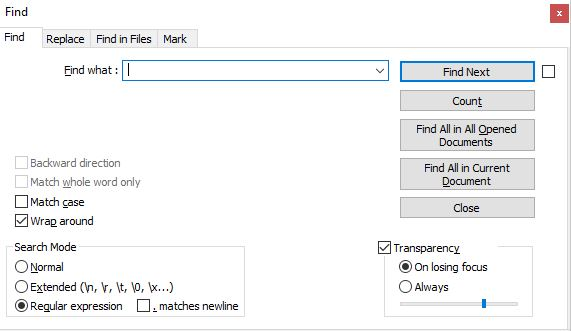## Removing extra digits after decimal and leave only two decimal places

`^(.*?\..?.?).*?\$`

your first match will leave the number with max 2 decimal digits.
So find with `^(.*?\..?.?).*?\$` and replace with `\1`

### Input

```27485.0375
75777.9125
10785.0125
13822.5
26700
41194.375
9375
4354.225
11564.475
12430.1
3246.4875
11596.3375
22466.0375
```

### Output:

```27485.03
75777.91
10785.01
13822.5
26700
41194.37
9375
4354.22
11564.47
12430.1
3246.48
11596.33
22466.03
```

## Extracting names from given list of URLs (using regex)

##### let us extract name form the URL
`https://www.google.com/name?=rajiv`
##### paste the URL in the notepad and press crtl+f , and select ‘regular expressions ‘ from search mode##### now, enter the regex in the find tab to fetch names
`.*?\?=(\w+)\$`

Now, the selected name will be fetched form regex as (\1).

it can work on numerous links like:-

```https://www.google.com/name?=rajiv/
http://www.example.com/asdas?=borde/id=?
http://www.example.com/asdasd?=bordex
http://www.example.com/name?=bordet
http://www.example.com/asdasd?=border
http://www.example.com/jsciloji?=bordeeeee
http://www.example.com/name?=bordee
http://www.example.com/name?=bordeeeeeee
http://www.example.com/09328e?=bordeeee
http://www.example.com/name?=borde
http://www.example.com/lpaosd?=borde
```

And only the part after first “?=” will be fetched

## Commonly used regular expressions (regex) for developers in PHP

Commonly used regular expressions (regex) for developers in PHP

Regex are used or can be used almost everywhere strings are involved. If you are dealing with text strings, you would often find patterns in them and you might be required to filter few of them based on some similarities and properties. For example: a currency or money is often written in this form –
[Currency symbol] [Digits] ([.] [Digits])maybe

Now, in order to filter any such things programmatically using a script, few of really helpful regular expressions are mentioned below:

1. Match 4 characters

```.{4}
or ....
```

2. Match first 4 characters

```^.{4}
```

3. Match last 3 characters

```.{3}\$
or
...\$
```

4. Match a digit

```[0-9]
```

5. Match an alphabet

```[a-zA-Z]
```

6. Match a digit occurring 0 or more times

```[0-9]*
```

7. Match a lowercase alphabet occurring 1 or more times

```[a-z]+
```

8. Match an uppercase alphabet either NOT occurring or occurring once ( 0 or 1 time)

```[A-Z]?
```

9. Match a character occurring exactly once
write the character itself in regex; similar to #4 and #5

10. Match a character literally (used to match meta-characters)
use \ before the character to be matched
If you want to find the occurrence of question mark(s) in a string
use \? (remember ? is used to find occurrence of at most 1 time but \? with find occurrence of ? itself exactly once)

Pro things

a regular expression to match an email address with 100% efficiency is really tough to be learnt here because according to RFC 0822
https://www.ietf.org/rfc/rfc0822.txt
, an email address can have letters like { } # \$ @ \ / – _ . alphabets numbers and more.

So, a quick regex for matching emails can be

```^[a-zA-Z0-9\._%\-^#\{\}][email protected]([a-zA-Z0-9.-]+\.)+[a-zA-Z]{2,9}\$
```

this will match {^}#@technacy.net which is an actual email address and is totally valid.

However this won’t work all the times, a more detailed regex would be something like this: (email addresses can end with IP addresses too)

`/^(?!(?:(?:\x22?\x5C[\x00-\x7E]\x22?)|(?:\x22?[^\x5C\x22]\x22?)){255,})(?!(?:(?:\x22?\x5C[\x00-\x7E]\x22?)|(?:\x22?[^\x5C\x22]\x22?)){65,}@)(?:(?:[\x21\x23-\x27\x2A\x2B\x2D\x2F-\x39\x3D\x3F\x5E-\x7E]+)|(?:\x22(?:[\x01-\x08\x0B\x0C\x0E-\x1F\x21\x23-\x5B\x5D-\x7F]|(?:\x5C[\x00-\x7F]))*\x22))(?:\.(?:(?:[\x21\x23-\x27\x2A\x2B\x2D\x2F-\x39\x3D\x3F\x5E-\x7E]+)|(?:\x22(?:[\x01-\x08\x0B\x0C\x0E-\x1F\x21\x23-\x5B\x5D-\x7F]|(?:\x5C[\x00-\x7F]))*\x22)))*@(?:(?:(?!.*[^.]{64,})(?:(?:(?:xn--)?[a-z0-9]+(?:-[a-z0-9]+)*\.){1,126}){1,}(?:(?:[a-z][a-z0-9]*)|(?:(?:xn--)[a-z0-9]+))(?:-[a-z0-9]+)*)|(?:\[(?:(?:IPv6:(?:(?:[a-f0-9]{1,4}(?::[a-f0-9]{1,4}){7})|(?:(?!(?:.*[a-f0-9][:\]]){7,})(?:[a-f0-9]{1,4}(?::[a-f0-9]{1,4}){0,5})?::(?:[a-f0-9]{1,4}(?::[a-f0-9]{1,4}){0,5})?)))|(?:(?:IPv6:(?:(?:[a-f0-9]{1,4}(?::[a-f0-9]{1,4}){5}:)|(?:(?!(?:.*[a-f0-9]:){5,})(?:[a-f0-9]{1,4}(?::[a-f0-9]{1,4}){0,3})?::(?:[a-f0-9]{1,4}(?::[a-f0-9]{1,4}){0,3}:)?)))?(?:(?:25[0-5])|(?:2[0-4][0-9])|(?:1[0-9]{2})|(?:[1-9]?[0-9]))(?:\.(?:(?:25[0-5])|(?:2[0-4][0-9])|(?:1[0-9]{2})|(?:[1-9]?[0-9]))){3}))\]))\$/iD`

So, what should be the ideal solution because you won’t like to check this^ regex all the time
Use PHP’s inbuilt filter – FILTER_VALIDATE_EMAIL
http://php.net/manual/en/filter.filters.validate.php

```if (!filter_var(\$email, FILTER_VALIDATE_EMAIL)) {   \$emailErr = "Invalid email format";  }
```

For security, w3schools mentioned passing and sanitizing all the data first with a function:

```function test_input(\$data) {   \$data = trim(\$data);   \$data = stripslashes(\$data);   \$data = htmlspecialchars(\$data);   return \$data; }
```

Matching a URL
A URL can have letters, digits, any character as a parameter or in host details. A simple version of regex to match http urls is

```^(http|https):\/\/((([a-z0-9.-]+\.)+[a-z]{2,4})|([0-9\.]{1,4}){4})(\/([a-zA-Z?-?0-9-_\?\:%\.\?\!\=\+\&\/\#\~\;\,\@]+)?)?\$
```

A detailed and more efficient regex:

`+// _^(?:(?:https?|ftp)://)(?:\S+(?::\S*)[email protected])?(?:(?!(?:10|127)(?:\.\d{1,3}){3})(?!(?:169\.254|192\.168)(?:\.\d{1,3}){2})(?!172\.(?:1[6-9]|2\d|3[0-1])(?:\.\d{1,3}){2})(?:[1-9]\d?|1\d\d|2\d|22[0-3])(?:\.(?:1?\d{1,2}|2[0-4]\d|25[0-5])){2}(?:\.(?:[1-9]\d?|1\d\d|2[0-4]\d|25[0-4]))|(?:(?:[a-z\x{00a1}-\x{ffff}0-9]-*)*[a-z\x{00a1}-\x{ffff}0-9]+)(?:\.(?:[a-z\x{00a1}-\x{ffff}0-9]-*)*[a-z\x{00a1}-\x{ffff}0-9]+)*(?:\.(?:[a-z\x{00a1}-\x{ffff}]{2,}))\.?)(?::\d{2,5})?(?:[/?#]\S*)?\$_iuS`

Diego Perini
https://gist.github.com/dperini/729294

However PHP’s inbuilt filter can also be used as the most ideal
use FILTER_VALIDATE_URL as we used FILTER_VALIDATE_EMAIL earlier

Match a Phone number
Regex for a phone number is easy if you want to match only MOBILE NUMBERS which must be of 10 digits and can include a country code like +91, 091, (91), (+91) or 0

```^(091|\+91|91|\(091\)|\(\+91\)|\(91\)|0)? ?[7-9][0-9]{9}\$﻿
```

https://regex101.com/r/kA6oD0/6

Match a Name
A name is supposed to have alphabets only but the length of first names and last names is not fixed or general at all. These lengths may vary thus the regex sounds funny at times.
^[a-zA-Z’ -]+\$

expression, match, php, regex

Clean html code with REGEX

```\$stripped = trim(preg_replace('/\s+/', ' ', \$sentence));
```